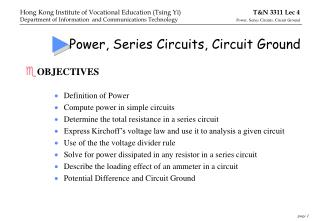DownloadDownload PresentationPower, Series Circuits, Circuit Ground

Power, Series Circuits, Circuit Ground

Télécharger la présentationPower, Series Circuits, Circuit Ground

- - - - - - - - - - - - - - - - - - - - - - - - - - - E N D - - - - - - - - - - - - - - - - - - - - - - - - - - -
Presentation Transcript

1. Power, Series Circuits, Circuit Ground • OBJECTIVES • Definition of Power • Compute power in simple circuits • Determine the total resistance in a series circuit • Express Kirchoff’s voltage law and use it to analysis a given circuit • Use of the the voltage divider rule • Solve for power dissipated in any resistor in a series circuit • Describe the loading effect of an ammeter in a circuit • Potential Difference and Circuit Ground

2. Notation of D.C. quantities • Direction of V and I • Source Load

3. Definition of Power

4. P is a measure of the rate of energy conversion

5. Example • Compute the power supplied to the electric heater shown below, using all three power formula.

6. SERIES CIRCUITS (1)

7. SERIES CIRCUITS (2)

8. SERIES CIRCUITS (3)

9. SERIES CIRCUITS (4) • Series connection of two resistors: • Prove the series resistance expression using the equivalent concept.

10. For the two resistors case, the voltage divider rule is: In general, for any number of resistors, the voltage drop across any resistor may be found as: Voltage Divider Rule

11. Ammeter Loading effect • Loading effect refers to those undesirable effects as a result of load insertion to a circuit. • When we use an ammeter to measure to measure the current of a circuit, the ammeter is inserted into the circuit in SERIES. • The ammeter will have a small resistance, Ra, this will alter the current to:

12. Potential difference • Potential difference (p.d.) (unit in volts) • P.d.is a voltage gradient between any two points in a circuit. (Current flows from high to low potential level) • P.d. across a passive component is called a voltage drop • P.d.s across R1 and R2 are the same (i.e. V1=V2) • Voltage at A (Vs) is twice voltage at B (V2) • P.d. is a vector quantity (mag. & direction) • P.d. is a relative magnitude • P.d. is always across two points • 0 volt is typically chosen as the reference voltage for a system or circuit.This is referred to as the GROUND.

13. Voltage measurement

14. Example of incorrect grounding technique One of the input of the voltmeter is grounded, an attempt to measure the non-grounded voltage between points A and B of the circuit results in short-circuiting point B to ground. The voltage value measured will be erroneous.